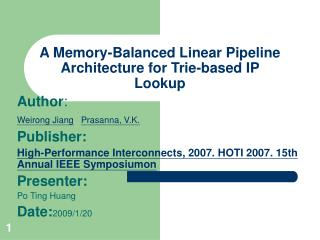Download PresentationA Memory-Balanced Linear Pipeline Architecture for Trie-based IP Lookup

# A Memory-Balanced Linear Pipeline Architecture for Trie-based IP Lookup

Download Presentation## A Memory-Balanced Linear Pipeline Architecture for Trie-based IP Lookup

- - - - - - - - - - - - - - - - - - - - - - - - - - - E N D - - - - - - - - - - - - - - - - - - - - - - - - - - -
##### Presentation Transcript

1. A Memory-Balanced Linear Pipeline Architecture for Trie-based IP Lookup Author: Weirong JiangPrasanna, V.K. Publisher: High-Performance Interconnects, 2007. HOTI 2007. 15th Annual IEEE Symposiumon Presenter: Po Ting Huang Date:2009/1/20

2. Introduction • All the (CAMP)(RING) Archetecture problems force us to rethink the linear pipeline architecture which has some nice properties • the linear pipeline can achieve a throughput of one lookup per clock cycle • support write bubbles for incremental updates without disrupting the ongoing pipeline operations • The delay to go through a linear pipeline is always constant, equal to the number of pipeline stages.

3. Ring • The resulting pipelines have multiple starting-points for the lookup process • This causes access conflicts in the pipeline and reduces throughput.~~(throughput degradation)

4. Ring architecture

5. CAMP • Using the similar idea but different mapping algorithm from RING • Unlike the Ring pipeline, CAMP has multiple entry stages and exit stages • ordering of the packet stream is lost • To manage the access conflicts between requests from current and preceding stages, several request queues are employed • A request queue results in extra delay for each request.~~(delay variation)

6. CAMP architecture

7. OLP(Optimized Linear Pipeline) • Constraint 1. All the subtries’ roots are mapped to the first stage. • offers more freedom to allow nodes on the same level of a subtrie to be mapped to different stages. • Constraint 2. If node A is an ancestor of node B in a subtrie, then A must be mapped to a stage preceding the stage B is mapped to

8. Partition

9. Partition • determine the initial stride which is used to expand the prefixes. • Given a leaf-pushed uni-bit trie, we use prefix expansion to split the original trie into multiple subtries • I: value of the initial stride which is used for prefix expansion; • P: number of pipeline stages; • T : the original leaf-pushed uni-bit trie; • T’ : the new trie after using I to expand prefixes in T ; • N(t): total number of nodes in trie t; • H(t): height of trie t.

10. Mapping • First we convert each subtrie to a segmented queue • Input each subtrie ~output a Queue • do a Breadth-First Traversal of each subtrie. Except the root of the subtrie ,each traversed node is pushed into the corresponding queue.

11. Next we pop the nodes from the queues and fill them into the memory of the pipeline stages. • sort Q1,Q2, · · ·,QS in the decreasing order of (1):NS(Qi) ≥ NS(Qj) (2):L(FS(Qi)) ≥ L(FS(Qj)) if NS(Qi)= NS(Qj) • We pop nodes until either there are Nr/Pr nodes in this stage, or no more frontend segments can be popped. • Once the current pipeline is full update FS(Qj), j = 1, 2, · · · , S;

12. STi: the ith subtrie, i = 1, 2, · · · , S; • SGi: the ith pipeline stage, i = 1, 2, · · ·, P; • Qi: the ith segmented queue, i = 1, 2, · · · , S; • FS(q): frontend segment3 of a segmented queue q, where q denotes any segmented queue. For example, in Figure 4 (c), FS(Q2) = {f, P6} ; • NS(q): number of segments in the segmented queue q; • L(q): length of queue q, i.e. the total number of nodes in queue q; • M(g): number of nodes mapped onto pipeline stage g; • Nr: number of remaining nodes to be mapped onto pipeline stages; • Pr: number of remaining pipeline stages for nodes to be mapped onto.

13. Mapping

14. Skip-enabling in the Pipeline • Each node stored in the local memory of a pipeline stage has two fields. Store the child node address. Store the distance to the pipeline stage • For example, when we search for prefix 111 in Stage 1, we retrieve (1) the node P8’s memory address in Stage 4, and (2) the distance from Stage 1 to Stage 4.

15. Performance

16. Performance

17. Performance • P: number of pipeline stages; • N: total number of trie nodes; • L: request queue size in CAMP.